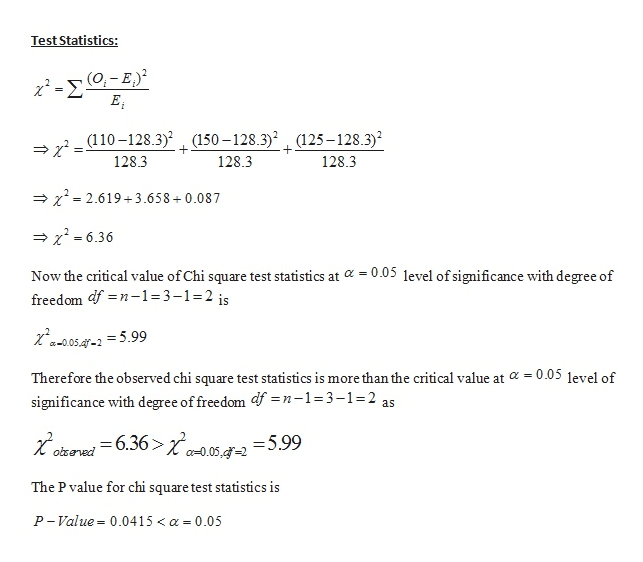# Using the data below, determine whether the proportion for each group is equal using a 5% significance level. Assume that all assumptions are satisfied.       Group  A.                   Group B.             Group C         110.                            150.                     125

Question
9 views

Using the data below, determine whether the proportion for each group is equal using a 5% significance level. Assume that all assumptions are satisfied.

Group  A.                   Group B.             Group C

110.                            150.                     125

check_circle

Step 1

According to the given question, to test whether the proportion for each group is equal using a 5% level of significance for the following data:

 Group Group -A Group -B Group- C Total Observed data 110 150 125 385 Expected data 128.3 128.3 128.3 385

We define the test hypothesis as:

Null hypothesis as:  the proportion for each group is equal

Against the alternative hypothesis as; the proportion for each group is not equal

In order to test this hypothesis we used chi square test statistics as follows:

Step 2help_outlineImage TranscriptioncloseTest Statistics: Σ Ei Sr=110-128.3) (150-128.3)2 (125-128.3) 128.3 + + 128.3 128.3 2.6193.658 + 0.087 x6.36 Now the critical value of Chi square test statistics at 0.05 level of significance with degree of freedom df n-1=3-1- 2 is Za-0.05.f-2=5.99 - 0.05 level of Therefore the observed chi square test statistics is more than the critical value at significance with degree of freedom df =n-1=3-1=2 as ,-6.36> a-.05,=2 =5.99 obseved The P value for chi square test statistics is P-Value= 0.0415 < a- 0.05 fullscreen

### Want to see the full answer?

See Solution

#### Want to see this answer and more?

Solutions are written by subject experts who are available 24/7. Questions are typically answered within 1 hour.*

See Solution
*Response times may vary by subject and question.
Tagged in

### Statistics